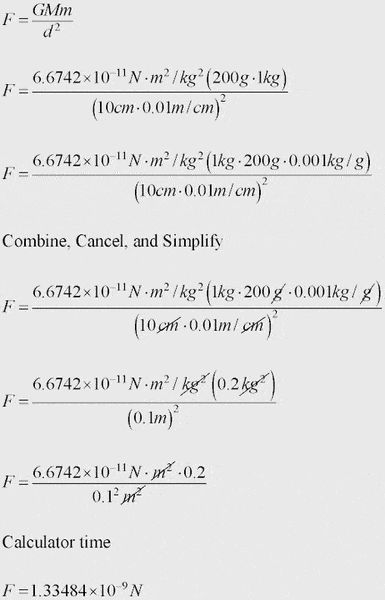# Unit for Gravitational Attraction Between Objects?

• Thrawn
In summary, the problem involves finding the gravitational attraction between two objects, one with a mass of 200g and the other with a mass of 1 kg, placed 10 cm apart. The gravitational force can be calculated using the formula F = G(mm)/d^2, where G is the universal gravitational constant. This value is 6.6742 x 10^-11*m^3*s^2*kg^2. The units for this value must be consistent with the units used for mass and distance in the problem. In this case, mass must be converted from grams to kilograms and distance must be in meters. Once all units are consistent, the calculation can be done using basic multiplication and division.

## Homework Statement

Two objects, one with a mass of 200g, and the other with a mass of 1 kg are placed 10 cm apart. What is the gravitational attraction between the objects?

Background: I'm performing an experiment in which a horizontal beam is suspended just above the ground with weights at either end. The larger weights are placed away from the smaller ones, so that the beam would have to turn for the distance between te large and small weights to decrease. I need to find the gravitational attraction between the objects, expressed in... well first I need to know which unit it would be expressed in.

I think it would be expressed in Newtons. Is this correct?

Yes, you are correct.

hage567 said:
Yes, you are correct.

Ok, so the formula F=G(mm)/d^2 would give the force in Newtons.

The gravitational force being 6.6742 x 10^-11*m^3*s^2*kg^2

So F= 6.6742 x 10^-11*m^3*s^2*kg^2 (200g*1000g)/ 0.01 ?
(200kg)/0.01

Which would give a force of... how do I calculate using G? Everything else I can do, but what do I need to do with G?

An example question would help, and no, this is not homework, seeing as the Ontario Grade 8 curriculum most certainly does not involve such mathematics.

G is not a force, it is called the universal gravitational constant. It is the same everywhere. For your calculation, you must convert all your units into kg, m, s, so everything is consistent. For example in your equation you have (200g*1000g). You need to change the mass from grams to kilograms, to be consistent with the units you see in G. The same goes for distance, it must be in meters.

Thrawn said:
Ok, so the formula F=G(mm)/d^2 would give the force in Newtons.

The gravitational force being 6.6742 x 10^-11*m^3*s^2*kg^2
These units are not quite right. It should be (N*m^2/kg^2) or (m^3/s^2*kg) if you expand it out.

So F= 6.6742 x 10^-11*m^3*s^2*kg^2 (200g*1000g)/ 0.01 ? this needs to be squared
(200kg)/0.01 I don't understand what this is.

Which would give a force of... how do I calculate using G? Everything else I can do, but what do I need to do with G?
You have a value for every variable in your equation, plug the numbers in and see what you get.

An example question would help, and no, this is not homework, seeing as the Ontario Grade 8 curriculum most certainly does not involve such mathematics.
It's not too difficult, just multiplication and division.

This is a good problem to get you used to unit manipulation. You did everything right, except as hage pointed out, you can't use grams because the number you are using for G only works with kilograms. I've cleaned up the math a bit so you can see step-by-step where different units combine and cancelLast edited: